# Visualizing with Pandas

Pandas uses Matplotlib under the hood. It provides some convenient functions for visualizing data. This built-in visualization capability is particularly useful for fast and easy visualizing of data series to facilitate data exploration.

import pandas as pd
import numpy as np
import matplotlib.pyplot as plt

%matplotlib notebook


Matplotlib comes with several built-in styles that allow us to change the look of our plots. These can be shown using the plt.style.available command.

plt.style.available

['seaborn-dark',
'seaborn-darkgrid',
'seaborn-ticks',
'fivethirtyeight',
'seaborn-whitegrid',
'classic',
'_classic_test',
'fast',
'seaborn-talk',
'seaborn-dark-palette',
'seaborn-bright',
'seaborn-pastel',
'grayscale',
'seaborn-notebook',
'ggplot',
'seaborn-colorblind',
'seaborn-muted',
'seaborn',
'Solarize_Light2',
'seaborn-paper',
'bmh',
'tableau-colorblind10',
'seaborn-white',
'dark_background',
'seaborn-poster',
'seaborn-deep']


The following sets the color pallete to use similar rgb values as the Tableau colorblind palatte, which uses blues and oranges preferentially.

plt.style.use('tableau-colorblind10')


The following uses the numpy cumsum function, which cumulatively sums up an array. The pandas date_range functions allows the index to be set to every day in 2017.

np.random.seed(123)

d = pd.DataFrame({'A': np.random.randn(365).cumsum(0),
'B': np.random.randn(365).cumsum(0) + 20,
'C': np.random.randn(365).cumsum(0) - 20},
index=pd.date_range('1/1/2017', periods=365))


A B C
2017-01-01 -1.085631 20.059291 -20.230904
2017-01-02 -0.088285 21.803332 -16.659325
2017-01-03 0.194693 20.835588 -17.055481
2017-01-04 -1.311601 21.255156 -17.093802
2017-01-05 -1.890202 21.462083 -19.518638
d.plot();

<IPython.core.display.Javascript object>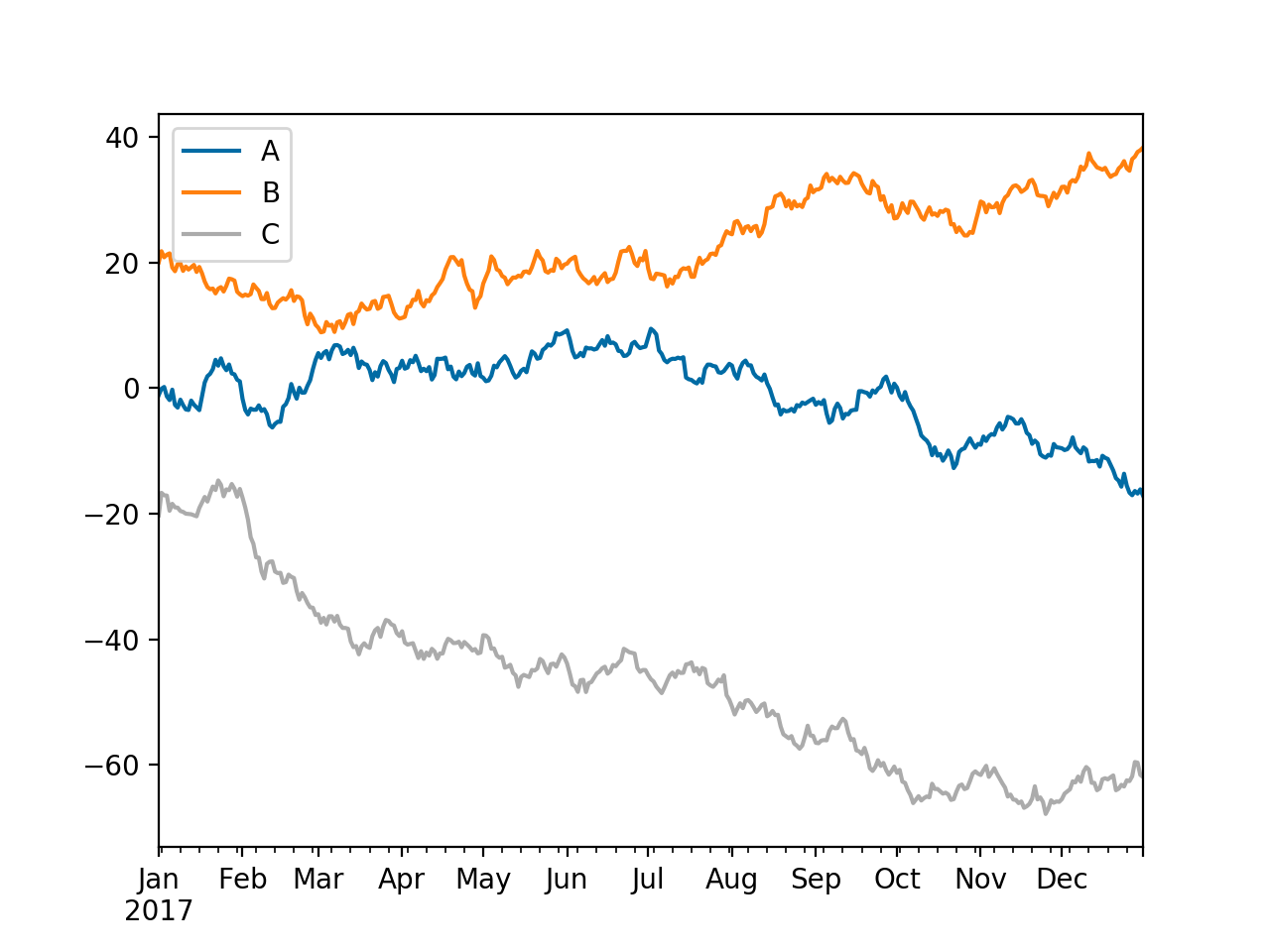Line plots are the default, but there are many kinds of plots that can be generated.

kind :

• 'line' : line plot (default)
• 'bar' : vertical bar plot
• 'barh' : horizontal bar plot
• 'hist' : histogram
• 'box' : boxplot
• 'kde' : Kernel Density Estimation plot
• 'density' : same as ‘kde’
• 'area' : area plot
• 'pie' : pie plot
• 'scatter' : scatter plot
• 'hexbin' : hexbin plot

This can be changed using the kind parameter, or by appending it to the plot command, such as d.plot.scatter. The following shows array B plotted against array A.

d.plot.scatter('A','B');

<IPython.core.display.Javascript object>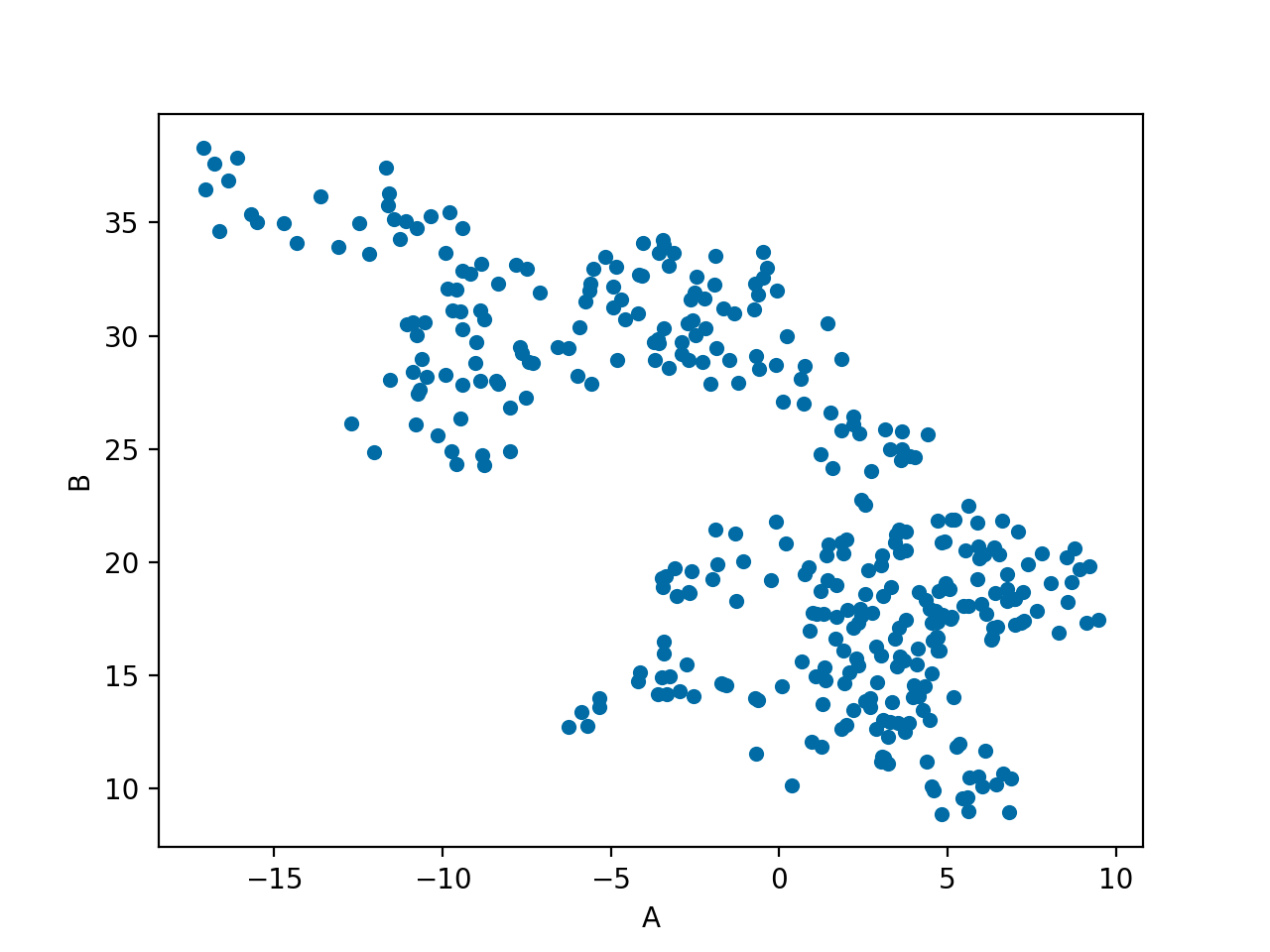The following shows C plotted against A, but with the color, c, and size, s, varying with B. Additionally, the colormap is set to viridis.

d.plot.scatter('A', 'C',
c='B',
s=d['B'],
colormap='viridis');

<IPython.core.display.Javascript object>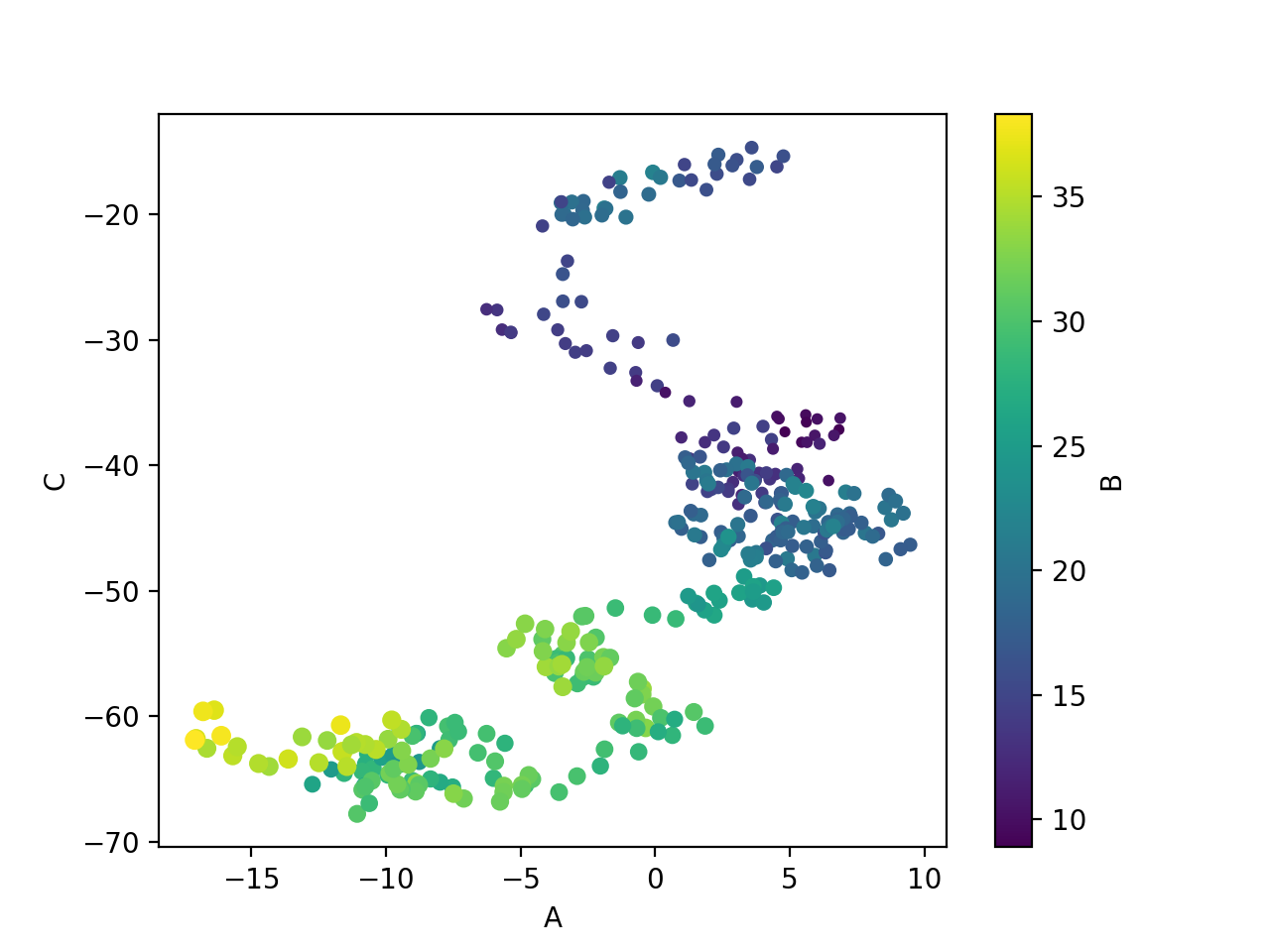plot.scatter returns a matplotlib.axes._subplot, so we can perform modifications on those items in the same manner as if we were using matplotlib. In the following, the plot is identical, except the aspect ratios are locked so that it is obvious to the viewer that the range of C is greater than the range of A.

ax = d.plot.scatter('A', 'C',
c='B',
s=d['B'],
colormap='viridis')
ax.set_aspect('equal')

<IPython.core.display.Javascript object>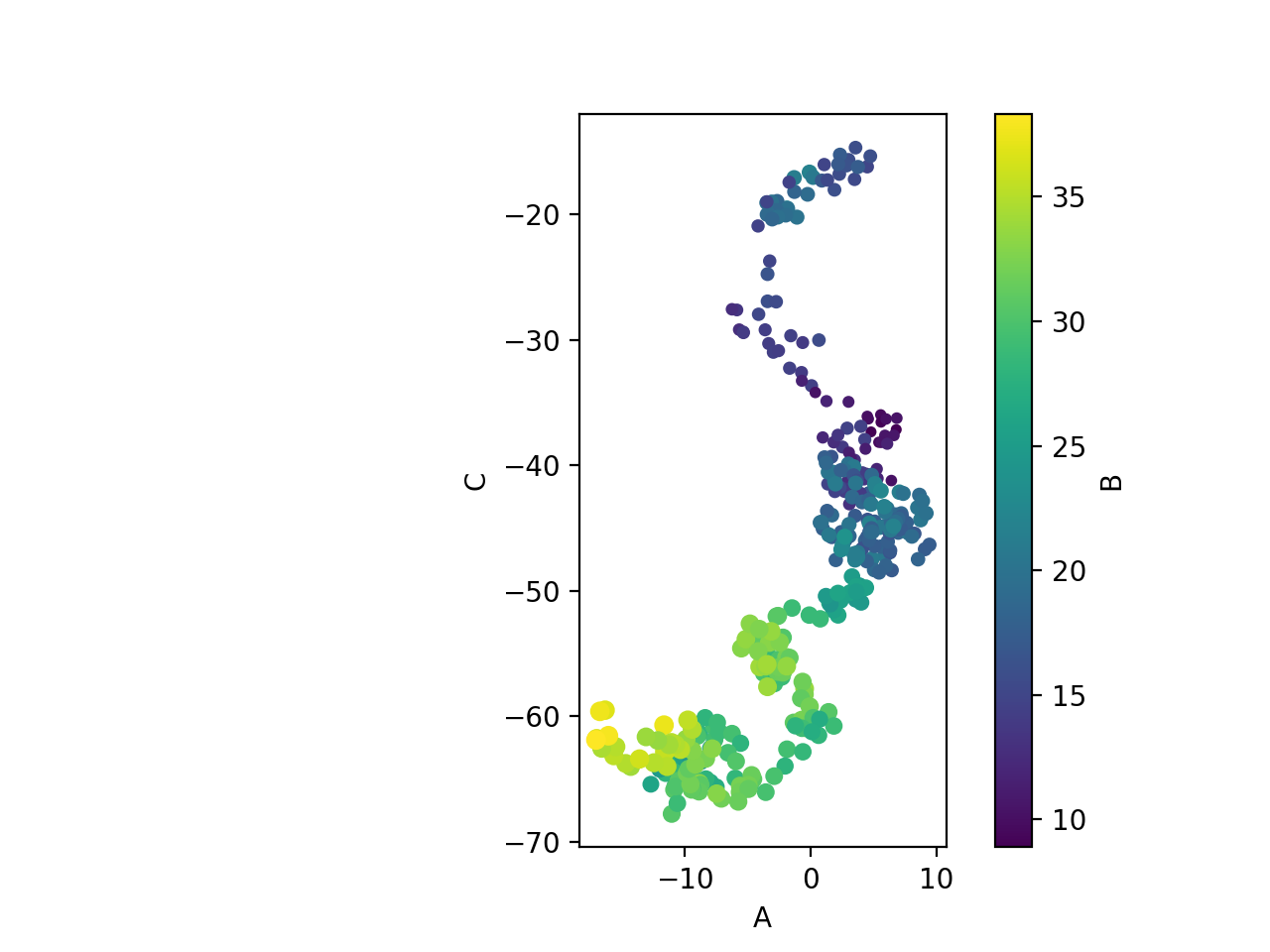Other examples of plots created using built-in Pandas visualization are shown below. Examples include boxplots, histograms, and kernel density estimate plots.

d.plot.box();

<IPython.core.display.Javascript object>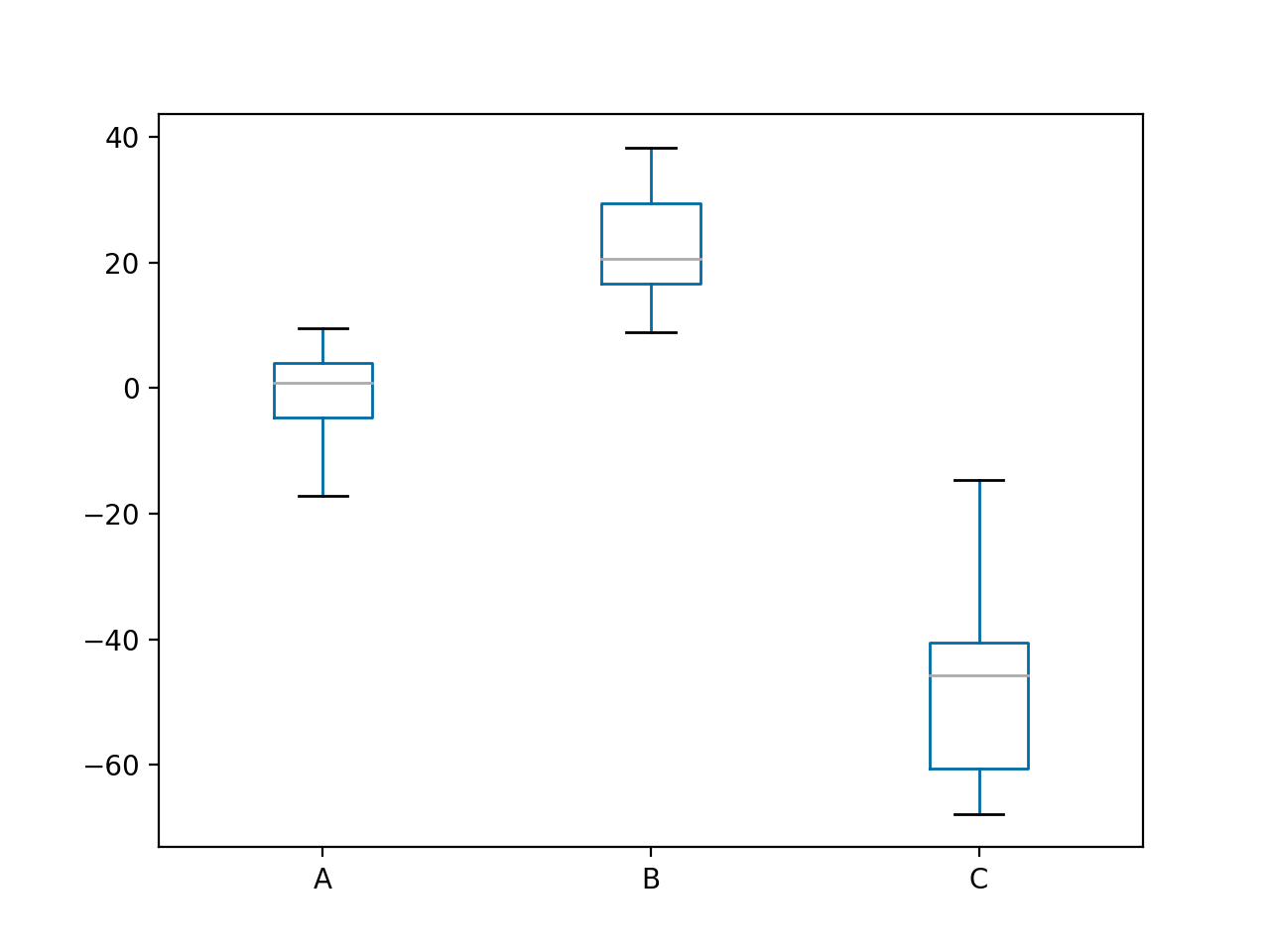d.plot.hist(alpha=0.7);

<IPython.core.display.Javascript object>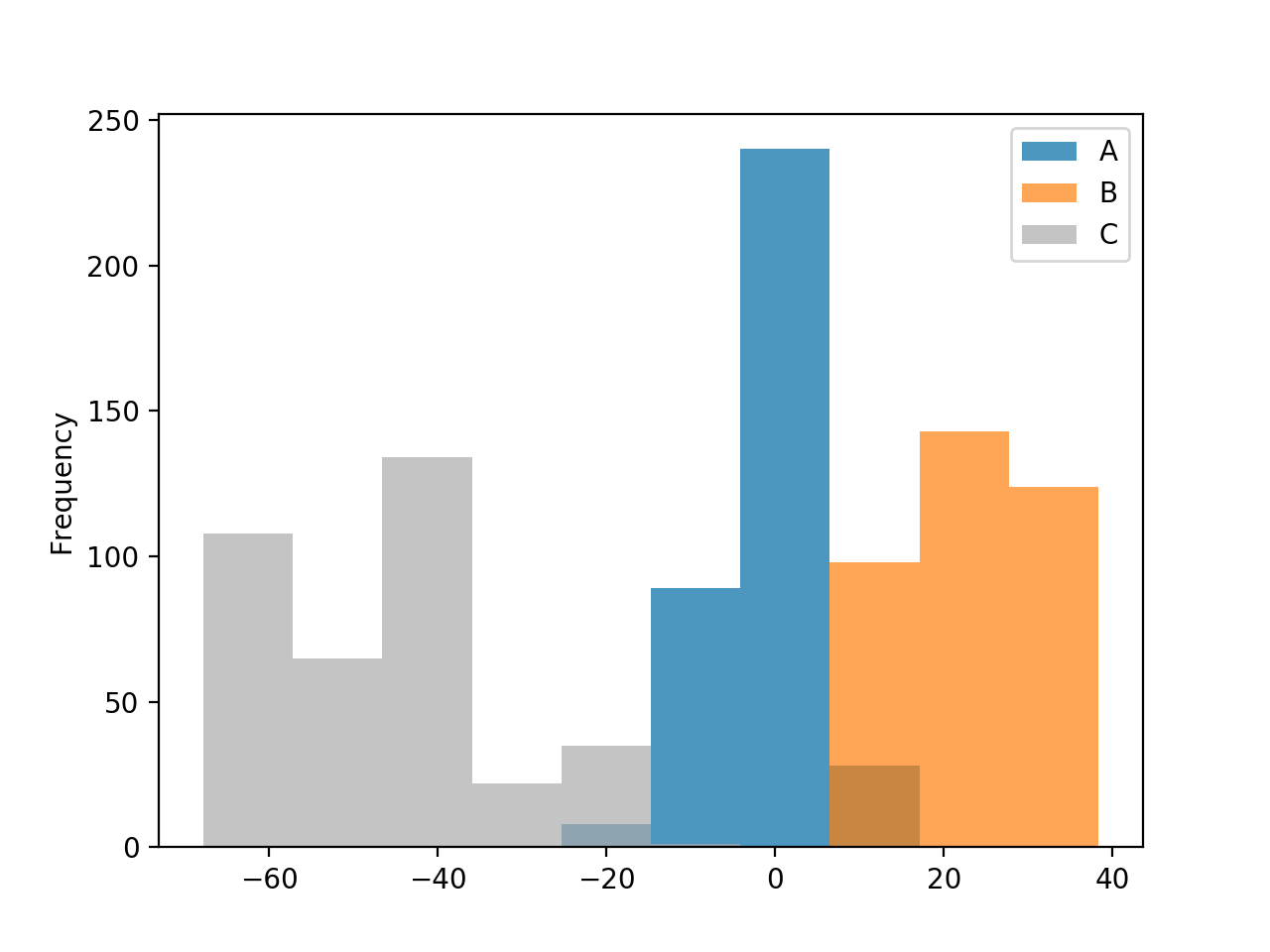d.plot.kde();

<IPython.core.display.Javascript object>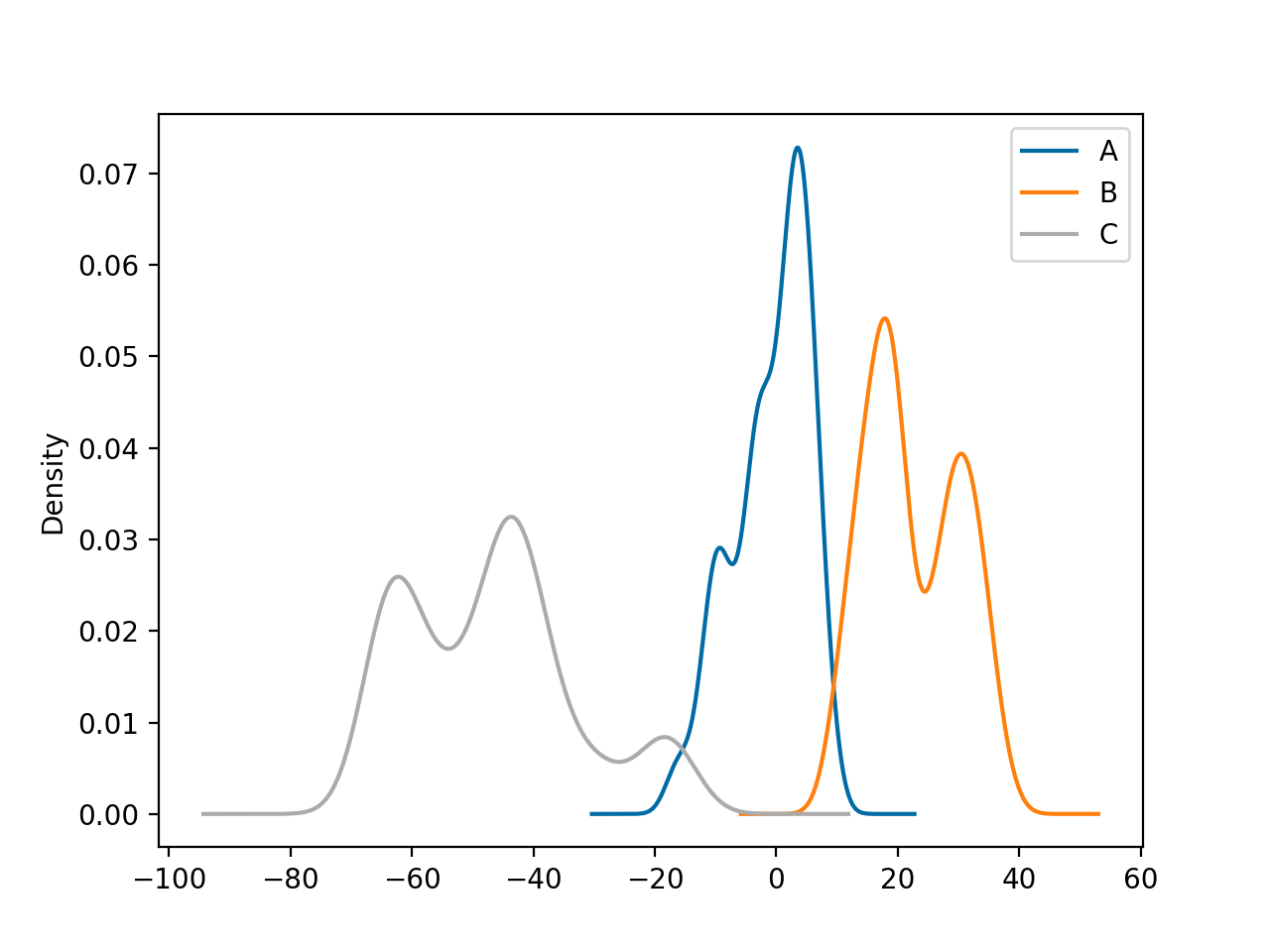Pandas also has plotting tools designed to help with visualizing large amounts of data or high dimensional data.

The iris dataset is a classic multivariate dataset that is built-in with seaborn. It contains the sepal length, sepal width, petal length, and petal width for hundreds of samples of 3 species of the iris flower.

import seaborn as sns



sepal_length sepal_width petal_length petal_width species
0 5.1 3.5 1.4 0.2 setosa
1 4.9 3.0 1.4 0.2 setosa
2 4.7 3.2 1.3 0.2 setosa
3 4.6 3.1 1.5 0.2 setosa
4 5.0 3.6 1.4 0.2 setosa

A scattermatrix is a way of comparing each column in a dataframe to every other column in a pairwise fashion. It is just a series of subplots with some plots shown as histograms and others scatterplots.

pd.plotting.scatter_matrix(iris);

<IPython.core.display.Javascript object>